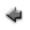previous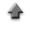home

# Scatter plot

You can draw a scatter plot of the data (x,y). To start, click "Scatter Plot and Fit" tab on the application.

If you use the sample data, click here, and save it in the appropriate directory (or folder).

When you draw the scatter plot of the sample data,

1. Click "Open data file", and specify the data file prepared according to the above instruction.
2. When clicking the "Plot" button bellow, the plot is displayed.

## Data

1. Each label for data can be set in the first row in the data file. The label can not include spaces. Each label has to be delimited by space or tab.
2. Data must be inputted by normal width.
3. Data must be inputted in the data file corresponding to the following data type.

### For Gaussian data

• When no errors are included in both x and y, the data must be inputted in order of x and y which are delimited by space or tab. Change the line, and input the data of the following row similarly until the input of the last data ends.
 x1 y1 x2 y2 . . . . . . xn yn
• When no errors are included in x and the errors are included in y, the data must be inputed in order of x, y and the error of y (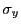) which are delimited by space or tab. Change the line, and input the data of the following row similarly until the input of the last data ends.
 x1 y1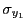x2 y2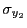. . . . . . . . . xn yn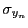• In addition, you can use the table data form in the data file. You may include the column data in the data file which are not used to plot. In that case, the specification of the columns in the data file used to plot is needed at the line "Data columns" in the following "Input" instruction.

### For Poisson data

• The data must be inputted in order of x and y which are delimited by space or tab. Change the line, and input the data of the following row similarly until the input of the last data ends.
 x1 y1 x2 y2 . . . . . . xn yn
• In addition, you can use the table data form in the data file. You may include the column data in the data file which are not used to plot. In that case, the specification of the columns in the data file used to plot is needed at the line "Data columns" in the following "Input" instruction.

## Inputs

1. Click "Open data file", and specify the data file prepared according to the above instruction.
2. When the labels for data are used, check the "Data labels" box.
3. The columns in the data file to be used to draw a plot can be specified at the line "Data columns". For instance, when x are put in the third column and y in the fifth column in the data file, specify "3 5", and check the "Data columns" box.
4. Check the "Gaussian" or "Poisson" according to the data type in the data set.
5. The range for x axis in the plot can be specified at the line "Data range". In that case, specify the range for x axis (the numerical values must be delimited by space), and check the "x range" box. For instance, when you want to assume the range for x to be 0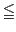x15, specify "0 15", and check the "x range" box.
6. The range for y axis in the plot can be specified at the line "Data range". In that case, specify the range for y axis (the numerical values must be delimited by space), and check the "y range" box. For instance, when you want to assume the range for x to be 0y15, specify "0 15", and check the "y range" box.
7. The title of the plot can be specified at the line "Graph title". Click the "Set" button after describing it.
8. The label of the abscissa in the plot can be specified at the line "Label of abscissa". Click the "Set" button after describing it.
9. The label of the ordinate in the plot can be specified at the line "Label of ordinate". Click the "Set" button after describing it.
10. The explanatory note (legend) of the plot can be specified at the line "Legend of data". Click the "Set" button after describing it.

## Outputs

1. The plot can be displayed when the "Plot" button is clicked.
2. A summary of the plot can also be displayed when the "Show data summary" button is clicked.

## Fitting a function to data

You can fit a function model to the plot.
1. Input a function model to be fitted at the line "Function f(x)", and click the "Set" button. About the input of the function, refer here.
2. For nonlinear function fitting, this fitting algorithm requires the specification of initial values of the parameters included in the function model. Then, you must input the initial values of the parameters at the line "Parameters(N)" which must be delimited by space, and click the "Set" button.
3. The range for x data to be fitted can be specified at the line "x data range". In that case, specify the range for x axis (the numerical values must be delimited by space), and check the "x data range" box. For instance, when you want to assume the range for x to be 0x15, specify "0 15", and check the "x data range" box.
4. Like the range for x data, the range for y data to be fitted can be specified at the line "y data range". In that case, specify the range for y in the plot (the minimum and the maximum values must be delimited by space), and check the "y data range". For instance, when you want to assume the range for y in the histogram to be 0y15, specify "0 15", and check the "y data range".
5. The explanatory note (legend) of the function can be specified at the line "Legend". Click the "Set" button after describing it.
6. When you use the second order derivatives of the function with respect to the parameters in computation of the curvature matrix elements and the parameter's errors, check the "2nd order derivatives(N)" box.
7. When clicking "Polynomial fit" or "Nonlinear fit" button, the summary of the fitting is displayed.
8. When the "Show error matrix" button is clicked, the elements of the error matrix is displayed.
9. When the "Show residual graph" button is clicked, the plot of the residuals is displayed.
10. When the "Show residuals" button is clicked, the data of the residuals is displayed.

Kazushi Neichi